Function Repository Resource:

# IsomorphicOrderedHypergraphQ

Determine whether two ordered (directed) hypergraphs are isomorphic

Contributed by: Jonathan Gorard
 ResourceFunction["IsomorphicOrderedHypergraphQ"][h1,h2] yields True if the ordered hypergraphs h1 and h2 are isomorphic, and False otherwise.

## Details and Options

Hypergraphs are specified as lists of hyperedges.
Two ordered hypergraphs are isomorphic if there exists a renaming of vertices that makes them identical.
The algorithm used in ResourceFunction["IsomorphicOrderedHypergraphQ"] is a direct generalization of Gorard’s uniqueness tree algorithm for standard graph isomorphism: https://arxiv.org/abs/1606.06399.

## Examples

### Basic Examples (3)

Determine that two ordered hypergraphs are isomorphic:

 In:=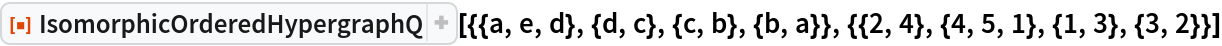Out=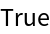Determine that two ordered hypergraphs are not isomorphic:

 In:=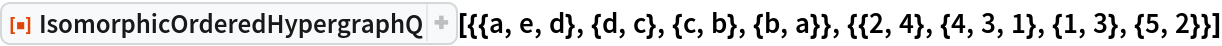Out=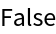Lists of hyperedges which are isomorphic as orderless hypergraphs may not be isomorphic as ordered hypergraphs:

 In:=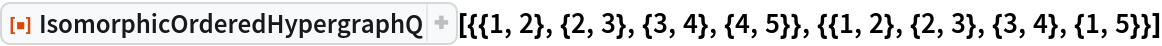Out=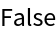### Scope (2)

IsomorphicOrderedHypergraphQ also works for standard directed graphs:

 In:=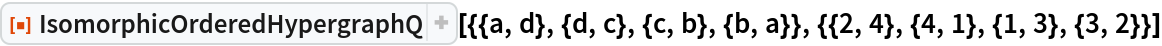Out=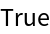In this case, it is functionally equivalent to IsomorphicGraphQ:

 In:=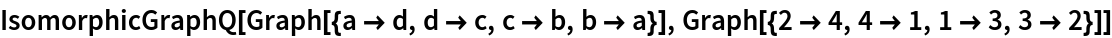Out=Furthermore, IsomorphicOrderedHypergraphQ supports isomorphism testing on ordered multihypergraphs (i.e. hypergraphs with ordered multihyperedges):

 In:=Out=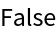Jonathan Gorard

## Version History

• 2.0.0 – 05 December 2019
• 1.0.0 – 01 November 2019